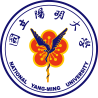# More2National Yang Ming University

\frac{d[protein of T7 polymerase ]}{dt}=RBS\times{PoPStrxC}\times\frac{N}{V}\times{a}\times{\frac{ [ROSoxyR]^{n1} }{ [Kd]^{n1}+[ROSoxyR]^{n1} }}}+PoPST7\times{T7 effect}\times\frac{N}{V}-{KdegT7}\times{T7}

In this equation, the production rate of T7 polymerase is composed of both synthesizing rate and degrading rate. RBS represents ribosome binding site strength, which is the affinity of ribosome to the starting site of mRNA; PoPS represents the promoter strength and \frac{[molecule]^{nmolecule}}{ Kdmolecule^{nmolecule}+[molecule]^{nmolecule} }, as mentioned above, represents the hill effect of transcription factors to the promoter.

For the section of the equation,\RBS\times{PoPStrxC}\times\frac{N}{V}\times{a}\times{\frac{ [ROSoxyR]^{n1} }{ [Kd]^{n1}+[ROSoxyR]^{n1} }}} represents the synthesizing rate of T7 polymerase under the influence of activator ROSoxyR complex, promoter trxC and terminators downstream; \PoPST7\times{T7 effect}\times\frac{N}{V}, similarly, represents the synthesizing rate of T7polymerase under the influence of T7promoter; \-{KdegT7}\times{T7}represents the natural degrading rate of T7 polymerase.